# Synchronous Machine

## Synchronous Machine on Infinite Bus Bars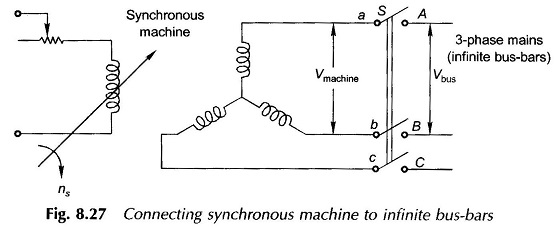Synchronous Machine on Infinite Bus Bars: A definite procedure has to be followed in connecting a Synchronous Machine on Infinite Bus Bars which for the present purpose will be assumed to be infinite. Infinite bus-bars means a 3-phase supply of constant voltage and frequency independent of the load exchanged (fed into the bus-bars or drawn …

## Armature Reaction in Synchronous Generator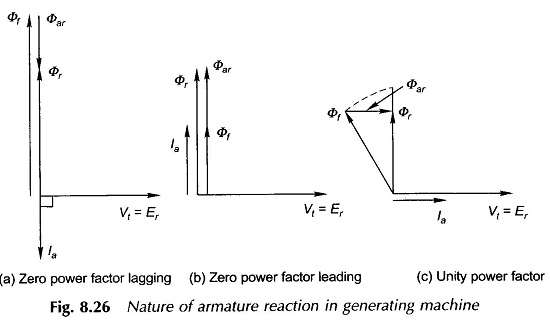Armature Reaction in Synchronous Generator: The Armature Reaction in Synchronous Generator is dependent on the power factor at which the machine is operating and upon the operating mode generating/motoring. For simplicity of explanation, it will be assumed here that the armature resistance and leakage reactance are negligible so that Figure 8.26 shows the phasor diagrams, …

## Armature Reaction in Synchronous Motor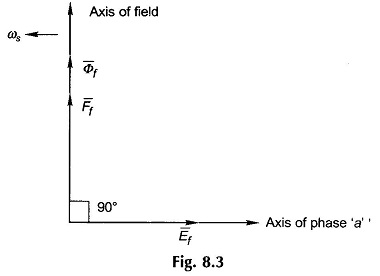Armature Reaction in Synchronous Motor: The Simple circuit model of the synchronous machine was obtained in Sec. 8.3 by making the assumption that the magnetic circuit of the machine is linear. However, it is known that, under normal operating conditions, the machine operates in a somewhat saturated region. In order to take account of magnetic …

## Determination of the Synchronous Reactance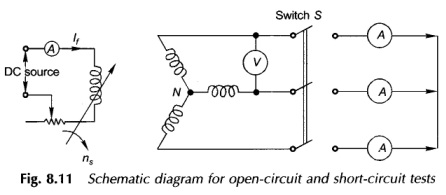Determination of the Synchronous Reactance | Open Circuit and Short Circuit test of Synchronous Machine: Determination of the Synchronous Reactance – With the assumption of a linear magnetic circuit, the circuit model (per phase) of a  synchronous machine is as given in Fig. 8.10c. If Ra is neglected, it then follows that It is immediately …

## Equivalent Circuit of Synchronous Motor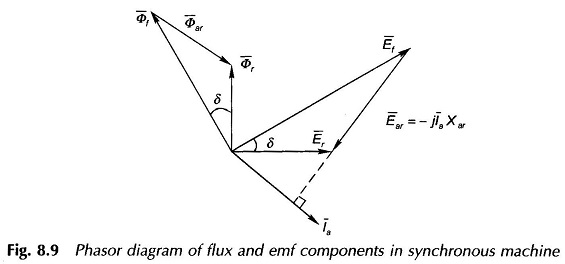Equivalent Circuit of Synchronous Motor: By assuming linearity of the magnetic circuit, it is possible to obtain simple Equivalent Circuit of Synchronous Motor. The validity of this assumption stems from the fact that air-gap is the predominant component of the magnetic circuit of the machine. As per Eq. (8.7), the resultant mmf phasor is given …

## Voltage Regulation of Synchronous Generator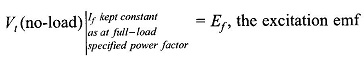Voltage Regulation of Synchronous Generator: The Voltage Regulation of Synchronous Generator is defined on lines similar to that of a transformer. Consider the generator supplying full-load current at a specified power factor and rated terminal voltage, Vt (rated). As the load is thrown off keeping the field current constant, the terminal voltage rises to The …

## Generating Mode and Motoring Mode of Synchronous Machine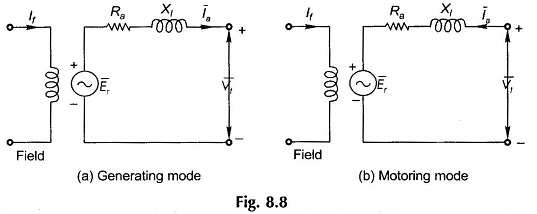Generating Mode and Motoring Mode of Synchronous Machine: Generating Mode and Motoring Mode of Synchronous Machine is derived by a realistic synchronous machine will have resistance ra and leakage reactance xl per armature phase which can be assumed to be lumped in series between the terminal voltage Vt and the air-gap emf Er for each …

## Construction of Cylindrical Rotor Synchronous Machine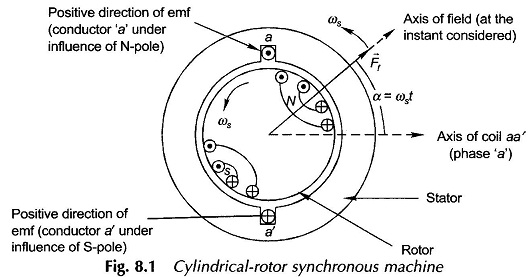Construction of Cylindrical Rotor Synchronous Machine: As stated earlier the Cylindrical Rotor Synchronous Machine offers constant permeance to mmf waves irrespective of the mechanical position of the rotor and is, therefore, simpler to model. Figure 8.1 shows the cross-sectional view of a 2-pole Cylindrical Rotor Synchronous Machine. The rotor has distributed windings which produce an …

## What is Synchronous Motor

What is Synchronous Motor | Construction | Advantages: A synchronous Motor is one of the important types of electric machines; in fact all generating machines at power stations are of synchronous kind and are known as synchronous generators or alternators. The structure and certain operational features of Synchronous Machine have already been explained in this …

Scroll to Top